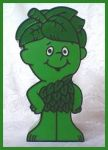# Music Quiz / Best Selling SPORCLE Albums

Random Music or Artist Quiz

## Can you name the best selling albums and their artists given only the letters in S-P-O-R-C-L-E?

#### bysproutcm  Plays Quiz Updated Mar 19, 2020

How to Play
Score
0/60
Timer
10:00
Artist
_ _ C _ _ E L    _ _ C _ S O _

P _ _ _    _ L O _ _

L E _    _ E P P E L _ _

_ C/_ C
_ E _ _    L O _ _
_ L E E _ _ O O _    _ _ C
_ L _ _ _ S    _ O R _ S S E _ _ E

_ _ E    _ E _ _ L E S

C E L _ _ E    _ _ O _
_ _ R _ _ _    C _ R E _
_ _ C _ S _ R E E _    _ O _ S
_ E E    _ E E S
_ R _ C E    S P R _ _ _ S _ E E _
_ _ R E    S _ R _ _ _ S
_ O _    _ O _ _

_ _ _ S    _ '    R O S E S

S P _ C E    _ _ R L S
_ R _ _ _ E _    S P E _ R S
C _ R O L E    _ _ _ _

S _ _ O _   &   _ _ R _ _ _ _ E L

_ _
_ C E    O _    _ _ S E

O _ S _ S

E _ _ _ E _
_ _ E L E
_ _ L L _    R _ _    C _ R _ S
C _ E R
_ E _    L E P P _ R _
P R _ _ C E   &   _ _ E    R E _ O L _ _ _ O _
_ S _ E R
Album
_ _ R _ L L E R
_ _ E    _ _ R _    S _ _ E    O _    _ _ E
_ O O _

'L E _   _ E P P E L _ _   _ _'
or   '_ O S O' or _ _ _ _ _ L E _

_ _ C _    _ _    _ L _ C _
_ _ _    O _ _    O _    _ E L L
R _ _ O _ R S
_ _ _ _ E _    L _ _ _ L E    P _ L L
S _ _.    P E P P E R ' S    L O _ E L _
_ E _ R _ S    C L _ _    _ _ _ _

L E _ ' S    _ _ L _    _ _ O _ _    L O _ E
_ _ S _ C    _ O _
_ _ L L E _ _ _ _ _
S P _ R _ _ S    _ _ _ _ _ _    _ L O _ _
_ O R _    _ _    _ _ E    _. S. _.
_ R O _ _ E R S    _ _    _ R _ S
S L _ P P E R _    _ _ E _    _ E _
_ P P E _ _ _ E    _ O R
_ E S _ R _ C _ _ O _

S P _ C E
…_ _ _ _    O _ E    _ O R E    _ _ _ E
_ _ P E S _ R _
_ R _ _ _ E    O _ E R    _ R O _ _ L E _
_ _ _ E R

_ _ E    _ O S _ _ _    _ R E E
_ _ E    S _ _ _
( _ _ _ _ ' S    _ _ E    S _ O R _ )
_ O R _ _ _ _    _ L O R _?

_ _ E    _ _ R S _ _ L L    _ _ _ _ E R S    L P
_ _
S O _ E    _ _ _ E    _ L L
_ E L _ E _ E
_ _ S _ E R _ _
P _ R P L E    R _ _ _
C O _ _ E S S _ O _ S

## From the Vault See Another

Drama TV by Episode Title

Why don't ya come over here and tell us more about these so-called episode titles?